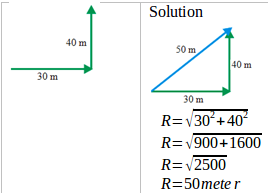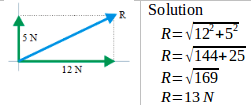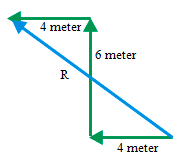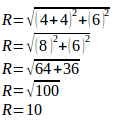# Determine resultant of two vectors using Pythagorean theorem

1. Determine the resultant of the two displacement vectors as shown in the figure below.[irp]

2. Find the resultant of two forces, 12 N and 5 N.[irp]

3. A student walks 4 meters to the west, then 6 meters to the north and 4 meters to the west. Find the student displacement.

SolutionDisplacement is 10 meter, to the northwest.

[irp]

[wpdm_package id=’542′]

[wpdm_package id=’554′]